# IBPS Clerk Pre Quantitative Aptitude Quiz- 2

## IBPS Clerk Pre Quantitative Aptitude Quiz

Quantitative Aptitude is a very essential and scoring section of almost every competitive exam. Candidates appear to be having difficulty with Quantitative Aptitude Questions, so they need to practice more. To help you prepare better, we are providing you with the IBPS Clerk Quantitative Aptitude Quiz. This IBPS Clerk Quantitative Aptitude Quiz is based on the latest and updated pattern. Candidates will be furnished with a detailed explanation for each question in this IBPS Clerk Quantitative Aptitude Quiz. This IBPS Clerk Quantitative Aptitude Quiz includes all types of questions that are generally asked in exams. This IBPS Clerk Quantitative Aptitude Quiz is absolutely free of cost. This IBPS Clerk Quantitative Aptitude Quiz will assist aspirants in achieving a good score in their upcoming examination.

Directions (1-5): In each of these questions, two equations (I) and (II) are given. You have to solve both the equations and give answer

1. I. 2x² + 11x + 15 = 0
II. 2y² + 15y + 27 = 0
(a) if x>y
(b) if x≥y
(c) if x<y
(d) if x ≤y

(e) if x = y or no relation can be established between x and y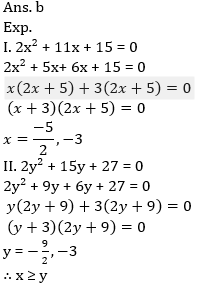2. I. x² – 40x + 384 = 0
II. y² – 25y + 150 = 0
(a) if x>y
(b) if x≥y
(c) if x<y
(d) if x ≤y
(e) if x = y or no relation can be established between x and y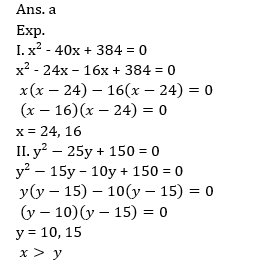3. I. 3x² – 10x – 125 = 0
II. y² – 21y + 108 = 0
(a) if x>y
(b) if x≥y
(c) if x<y
(d) if x ≤y
(e) if x = y or no relation can be established between x and y4. I. 5x² + 33x + 54 = 0
II. 3y² + 22y + 35 = 0
(a) if x>y
(b) if x≥y
(c) if x<y
(d) if x ≤y
(e) if x = y or no relation can be established between x and y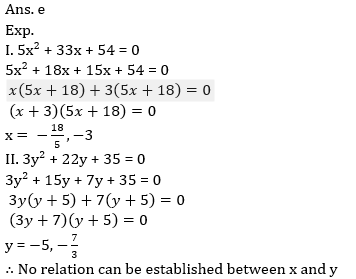5. I. 2x + 7y = 13
II. 6x +y = 219
(a) if x>y
(b) if x≥y
(c) if x<y
(d) if x ≤y
(e) if x = y or no relation can be established between x and y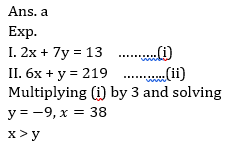6. 12 men and 16 women can do a piece of work in 5 days while 13 men and 24 women can do same piece of work in 4 days. How long will 7 men and 10 women take to do same piece of work.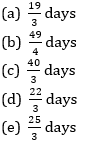7. In a box there are seven honor 7x mobile and five one plus five mobiles. A man was asked to select two mobiles randomly. What is probability that man select at least one ‘One plus five’ mobile?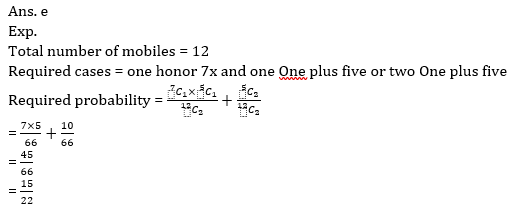8. A man had two sons and one daughter. To the elder son he gave 4/9 of his property, to the younger son 20% of the remainder and rest to his daughter. Find the share of the younger son if daughter gets Rs. 5200.
(a) 5,200
(b) 1,200
(c) 1,300
(d) 3,900
(e) 2,6009. The C.I. earned on an amount of Rs. 25500 at the end of three years is Rs. 8440.50. What would be the simple interest earned on the same amount at the same rate in the same period.
(a) Rs.4650
(b) Rs.4590
(c) Rs.6120
(d) Rs.7650
(e) Rs.9180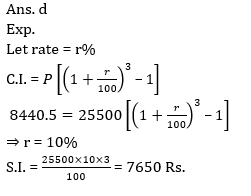10. A two-digit number, 20% more than the number obtained after reversing its digits. Find the original number.
(a) 45
(b) 36
(c) 63
(d) 54
(e) 21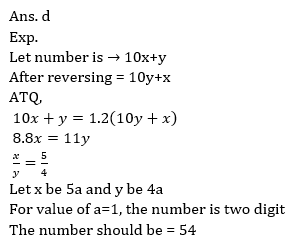###Click to Buy Bank MahaCombo PackageRecommended PDF’s for:

#### Most important PDF’s for Bank, SSC, Railway and Other Government Exam : Download PDF Now

AATMA-NIRBHAR Series- Static GK/Awareness Practice Ebook PDF Get PDF here
The Banking Awareness 500 MCQs E-book| Bilingual (Hindi + English) Get PDF here
AATMA-NIRBHAR Series- Banking Awareness Practice Ebook PDF Get PDF here
Computer Awareness Capsule 2.O Get PDF here
AATMA-NIRBHAR Series Quantitative Aptitude Topic-Wise PDF Get PDF here
AATMA-NIRBHAR Series Reasoning Topic-Wise PDF Get PDF Here
Memory Based Puzzle E-book | 2016-19 Exams Covered Get PDF here
Caselet Data Interpretation 200 Questions Get PDF here
Puzzle & Seating Arrangement E-Book for BANK PO MAINS (Vol-1) Get PDF here

3